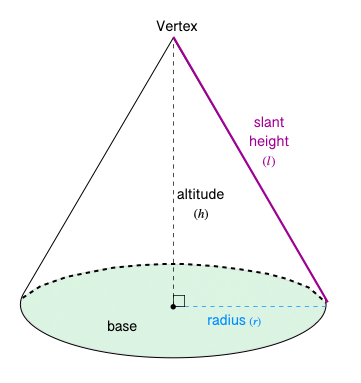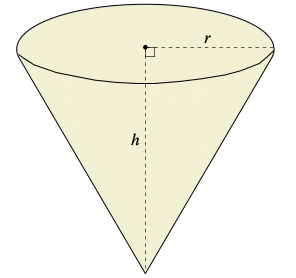407 493 6601

## Introduction

• A cone is a three-dimensional (solid) figure having a circular base and a pointed edge at the top called the vertex.
• A right circular cone is similar to a regular pyramid except that its base is a circle.• The altitude of a right circular cone $\left(h\right)$ is the measure of the perpendicular line segment joining the vertex and the center of the base.
• The slant height $\left(l\right)$ of a right circular cone is the measure of the line segment joining the vertex and any point on the circumference of the base.
• The mathematical relationship between the altitude, the radius of the base, and slant height is given by: $l=\sqrt{{r}^{2}+{h}^{2}}$.
• The area of the base is $B={\mathrm{\pi r}}^{2}$.

## Volume of a Cone

• The volume (capacity) of a cone is the amount of space occupied by it in a three-dimensional plane.
• The volume of a cone is measured in cubic units.
• If we know the radius of the base and altitude of a right circular cone, its volume can be calculated using the formula mentioned below.$Volum{e}_{cone}=\frac{1}{3}{\mathrm{\pi r}}^{2}\mathrm{h}$ where $\mathrm{\pi }=\frac{22}{7}\approx 3.14$$r=$the radius of the base, and $h=$altitude

## Surface Area of a Cone

• There are two types of surface areas associated with a right circular cone.

1. Lateral Area: It is the region occupied by the curved surface of the right circular cone.

$L{A}_{cone}=\mathrm{\pi rl}$, where $r=$the radius of the base, and $l=$slant height

2. Total Area: It is the total area occupied by a right circular cone, including the base area.

$T{A}_{cone}=L{A}_{cone}+B$

$=\mathrm{\pi r}\left(\mathrm{l}+\mathrm{r}\right)$, where $B=$the area of the base

## Solved Examples

Question 1: Find the volume of a right circular cone if the radius of its base is 4 cm and its altitude is 15 cm.

Solution: $Volum{e}_{cone}=\frac{1}{3}{\mathrm{\pi r}}^{2}\mathrm{h}$

$=\frac{1}{3}\mathrm{\pi }×{\left(4\right)}^{2}×15$

Question 2: Find the lateral area of a right circular cone in terms of $\mathrm{\pi }$ if the radius of its base is 3 ft and the altitude is 4 ft.

Solution: First we need to find the measure of slant height.

$l=\sqrt{{r}^{2}+{h}^{2}}$$=\sqrt{{\left(3\right)}^{2}+{\left(4\right)}^{2}}$$=\sqrt{25}$

$L{A}_{cone}=\mathrm{\pi rl}$

$=\pi ×3×5$

Question 3: Find the total surface area of a right circular cone if radius is 3 m and the slant height is15 m.

Solution: $T{A}_{cone}=\mathrm{\pi r}\left(\mathrm{l}+\mathrm{r}\right)$

$=\pi ×3\left(15+3\right)$

$=\pi ×3×18$

## Cheat Sheet

• Area of the base, $B={\mathrm{\pi r}}^{2}$
• Relation between the radius of the base, height, and slant height: $l=\sqrt{{r}^{2}+{h}^{2}}$
• $Volum{e}_{cone}=\frac{1}{3}{\mathrm{\pi r}}^{2}h$
• Lateral Surface Area, $L{A}_{cone}=\mathrm{\pi rl}$
• Total Surface Area, $T{A}_{cone}=L{A}_{cone}+B$$=\mathrm{\pi r}\left(\mathrm{l}+\mathrm{r}\right)$

## Blunder Areas

• The volume of the cone = $\frac{1}{3}$volume of the cylinder.
• All the formulas mentioned in the above sections are applicable to right circular cones only.